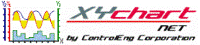XY Chart NET 3 Control Reference
MaxXValue Property

Use this property to obtain the current maximum x-value, of a specific x-axis, displayed on the graph.  The value returned is of type Double.

Syntax
 [Visual Basic] ReadOnly Property MaxXValue(XAxisNumber As Integer) As Double [C#] double XYChartNETCtl.get_MaxXValue(int XAxisNumber) [C++] property double MaxXValue[int]

Parameters
XAxisNumber
The x-axis index to obtain the current maximum value of.  Valid values are 0..(NumXScales - 1).
Remarks

This property can be used to obtain the current maximum x-value displayed on the graph after a zoom-in has occurred.

Example
```' XYChartNETCtl1 is the name of the XY Chart NET control instance placed on the form.

Dim tmpMin As Double
Dim tmpMax As Double

tmpMin = XYChartNETCtl1.MinXValue(3)  'Get the current min value for x-axis 3
tmpMax = XYChartNETCtl1.MaxXValue(3)  'Get the current max value for x-axis 3
```
```// XYChartNETCtl1 is the name of the XY Chart NET control instance placed on the form.

double tmpMin;
double tmpMax;

tmpMin = XYChartNETCtl1.get_MinXValue(1);  //Get the current min value for x-axis 1
tmpMax = XYChartNETCtl1.get_MaxXValue(1);  //Get the current max value for x-axis 1
```
```// XYChartNETCtl1 is the name of the XY Chart NET control instance placed on the form.

double tmpMin;
double tmpMax;

tmpMin = XYChartNETCtl1->MinXValue;  //Get the current min value for x-axis 0
tmpMax = XYChartNETCtl1->MaxXValue;  //Get the current max value for x-axis 0
```